# What Is VDC Voltage?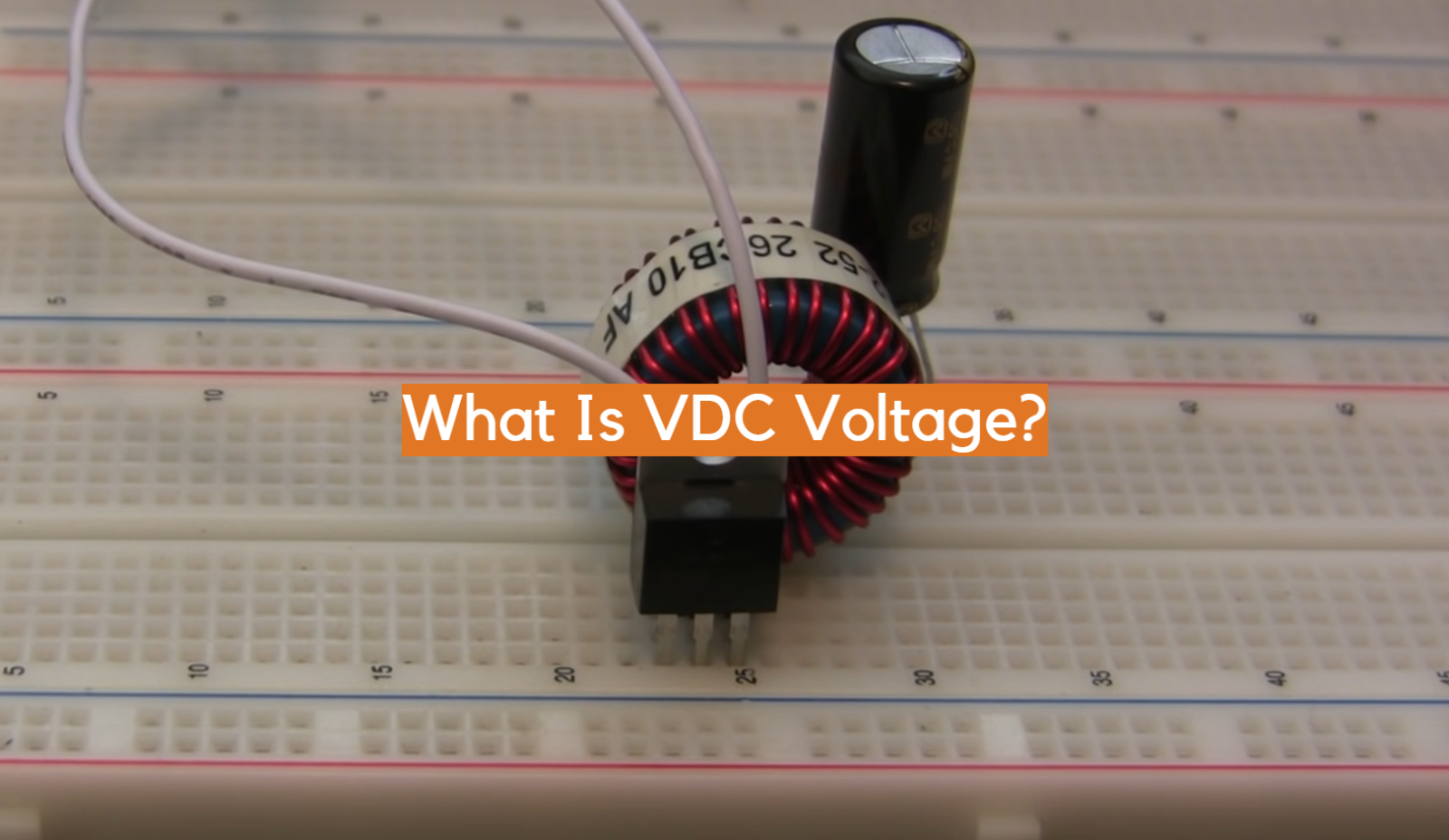Voltage is a fundamental concept in electrical engineering, and it plays a crucial role in powering electronic devices and systems. VDC voltage, also known as Volts Direct Current, refers to the magnitude of direct current voltage.

Unlike alternating current (AC), which periodically changes direction, direct current (DC) flows in one direction only. VDC voltage is commonly found in various applications, from small electronic devices to automotive systems.

Understanding the characteristics and applications of VDC voltage is essential for anyone working with electrical circuits or seeking to comprehend the principles behind DC-powered devices. In this article, we will delve into the details of VDC voltage, its significance, and how it differs from other forms of voltage.

## What are DC and AC Voltage?

Electricity is a fundamental aspect of our daily lives, powering everything from our electronic devices to the lighting in our homes. To understand electricity better, it is essential to grasp the concepts of DC (Direct Current) and AC (Alternating Current) voltage. These terms refer to the two different types of electrical currents that exist.

DC Voltage, as the name suggests, refers to the flow of electrical current in one direction only. It maintains a constant polarity and magnitude, without changing its direction . This type of current is commonly found in batteries and other sources of stored electrical energy. When you connect a battery to a device, the current flows from the positive terminal to the negative terminal, creating a steady flow of electrons.

On the other hand, AC Voltage represents an electrical current that periodically changes its direction and magnitude. It is the type of current typically provided by power grids and used in household outlets. Alternating Current alternates between positive and negative polarities, rapidly switching direction. This rapid oscillation allows power to be transmitted efficiently over long distances, making it the preferred choice for large-scale electrical distribution.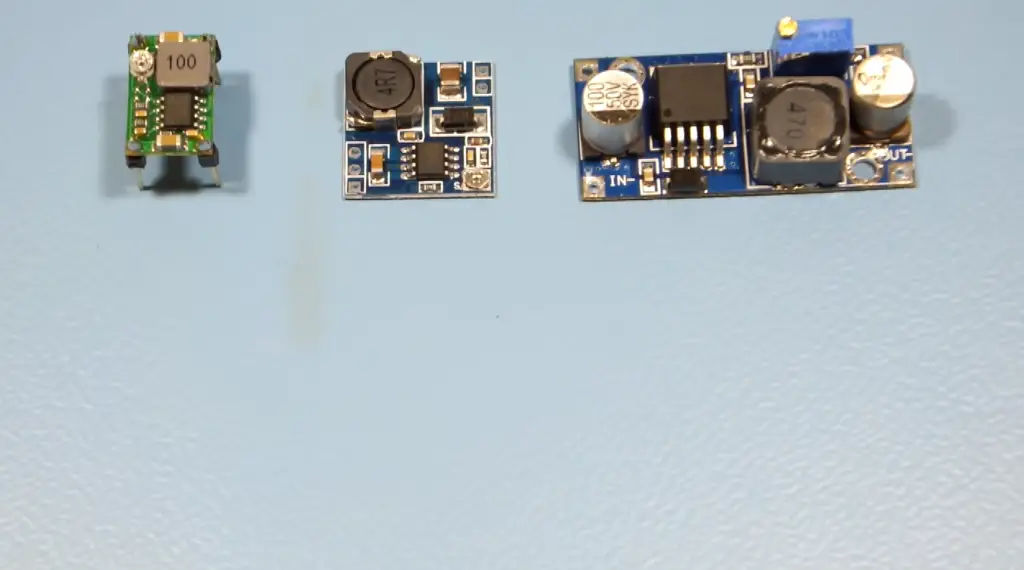## What Is VAC Voltage?

VAC Voltage, also known as AC Voltage, stands for Volts Alternating Current. It is a measurement that describes the magnitude of an alternating current. VAC Voltage is commonly seen on electrical devices, indicating the range of alternating current they can handle or require to operate effectively. For example, you might find a label on a laptop charger stating “Input: 100-240VAC”, indicating that the charger can accept a range of voltages in the alternating current format.

## What Is VDC Voltage?

VDC Voltage, also referred to as DC Voltage, stands for Volts Direct Current. It represents the magnitude of a direct current, indicating the amount of electrical potential difference between two points in a circuit that allows the flow of electrons in a single direction. VDC Voltage is crucial in powering devices that operate on direct current, such as electronic circuits and components like microcontrollers or integrated circuits.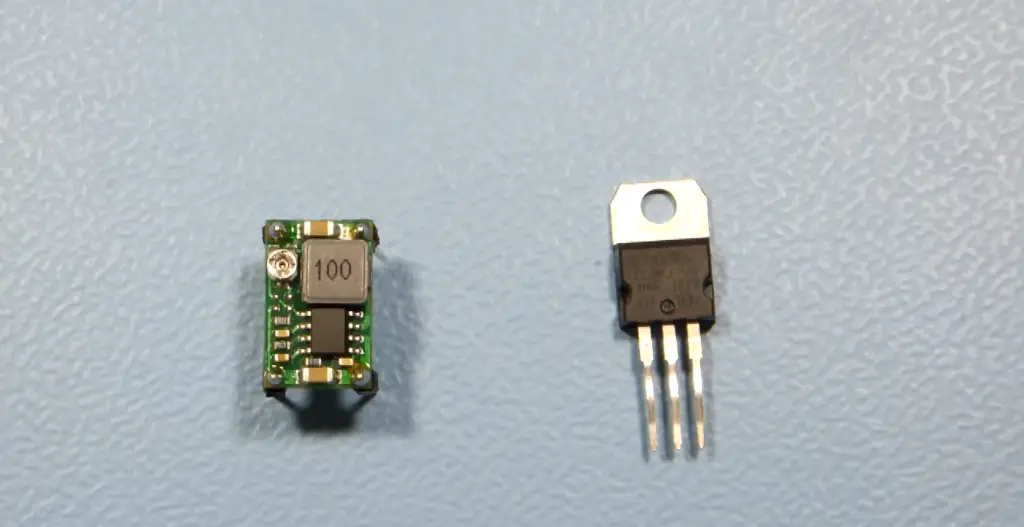## Are DC And VDC the Same?

In simple terms, DC and VDC are essentially the same. Both terms refer to direct current voltage, which represents the unidirectional flow of electrical charge. The only difference lies in the notation used to represent this voltage. DC is a general term encompassing all direct current, while VDC is a more specific notation indicating voltage in volts.

## Are VAC And Voltage the Same?

VAC and Voltage are not the same, but they are related terms. Voltage is a broader term that encompasses both AC and DC voltages. It refers to the electrical potential difference between two points in a circuit, regardless of whether it is alternating or direct current. On the other hand, VAC specifically denotes the magnitude of an alternating current.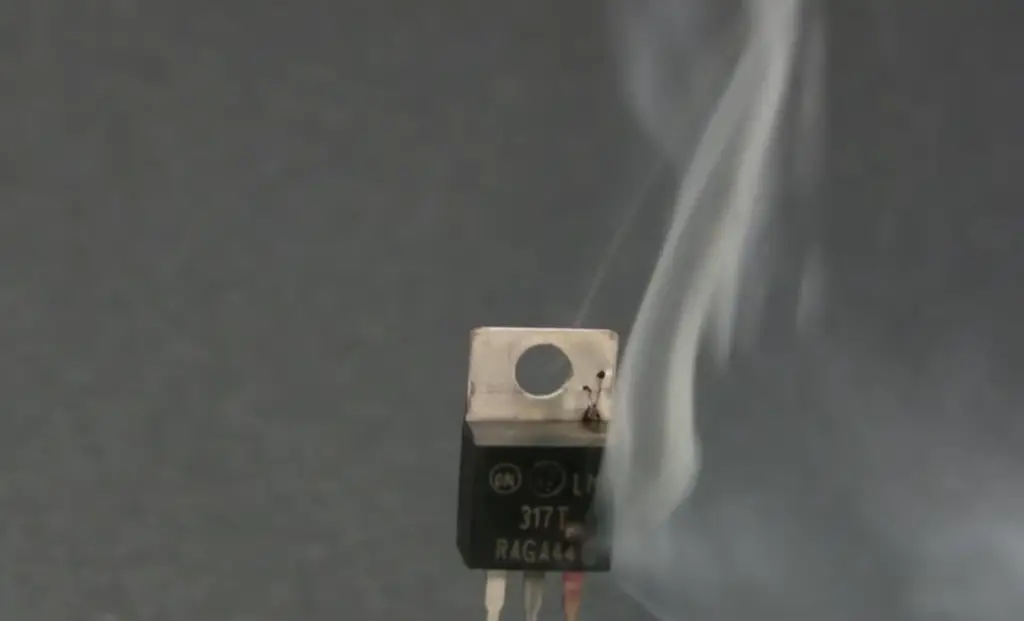## The VDC Output Power Supply

In various electronic applications, a stable and reliable source of direct current power is required. This is where VDC output power supplies come into play. These power supplies convert an input voltage, which may be AC or DC, into a regulated output voltage of direct current .

## VDC Output Power Supply Types:

• Linear power supplies operate by using a linear regulator to regulate the output voltage. They are known for their simplicity, low cost, and relatively low noise. However, they are less efficient compared to other types and may produce more heat;
• Switching power supplies, on the other hand, use switching regulators to regulate the output voltage. They are known for their high efficiency, compact size, and ability to handle a wide range of input voltages. Switching power supplies are commonly found in electronic devices like computers, televisions, and mobile phones
• Battery-based power supplies, as the name suggests, use rechargeable batteries as their power source. These power supplies are portable and provide a reliable source of direct current when other power sources are not available;

## VDC Voltage Versus VAC Voltage

The distinction between VDC and VAC voltage lies in the type of current they represent. VDC voltage refers to direct current, which flows in one direction, while VAC voltage represents alternating current, which periodically changes direction. The choice between VDC and VAC voltage depends on the specific requirements of the device or application being powered.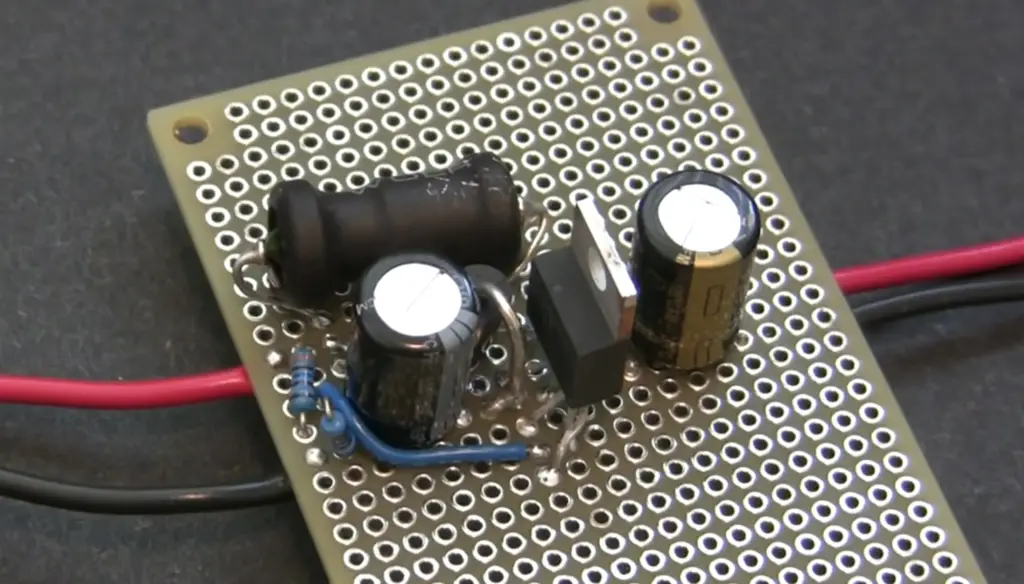## How To Calculate VDC From VAC?

Converting VAC voltage to VDC voltage requires a rectifier circuit. A rectifier is an electrical device that converts alternating current into direct current. It typically consists of diodes that allow the flow of current in one direction while blocking it in the opposite direction.

To calculate VDC from VAC, follow these steps:

Determine the peak voltage (Vpeak) of the AC signal. This is the maximum voltage value in the AC waveform.

Calculate the peak-to-peak voltage (Vpp) by multiplying Vpeak by 2. Vpp represents the difference between the maximum positive and negative voltage values.

Use the following formula to calculate the RMS (Root Mean Square) voltage (Vrms):

Vrms = Vpp / √2

The RMS voltage represents the equivalent DC voltage that would produce the same amount of power as the AC voltage.

Finally, the calculated Vrms value represents the VDC voltage .

It’s important to note that this calculation assumes a perfect sinusoidal waveform for the AC voltage. In practice, the waveform may deviate from a perfect sinusoid, and additional considerations may be necessary.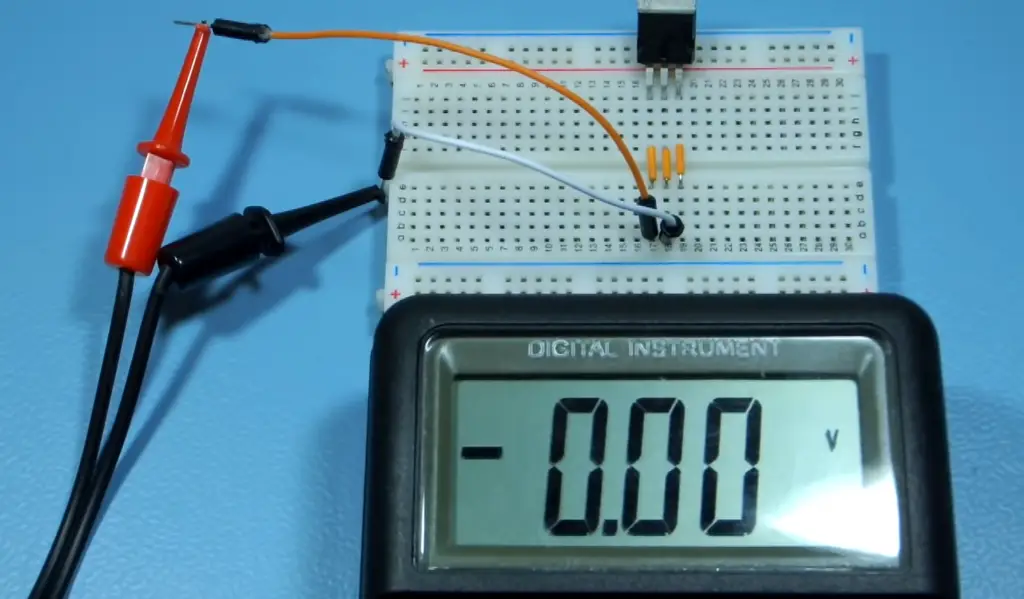## FAQ:

### 1. Can you use a VAC switch for DC?

No, you cannot use a VAC (Volts Alternating Current) switch for DC (Direct Current) applications. VAC switches are specifically designed to handle and interrupt the flow of alternating current, which has different characteristics compared to direct current. Direct current flows in one direction only, whereas alternating current periodically changes its direction .

The design and construction of switches are tailored to the specific requirements of the current type they are intended for. Therefore, using a VAC switch for DC can result in improper operation, potential damage to the switch, and safety hazards.

### 2. Are volts and VDC the same?

Volts (V) and VDC (Volts Direct Current) are closely related but not exactly the same. Volts represent the unit of measurement for voltage, which is the electrical potential difference between two points in a circuit. It is a general term that encompasses both AC and DC voltages.

On the other hand, VDC specifically refers to the magnitude of direct current voltage, indicating the amount of electrical potential difference between two points in a circuit that allows the flow of electrons in a single direction. So while volts are a broader term, VDC is a more specific notation indicating direct current voltage.

### 3. How do you calculate VDC voltage?

To calculate VDC voltage, you need to measure the direct current voltage using a voltmeter or multimeter.

Connect the positive (red) probe of the meter to the positive terminal of the voltage source and the negative (black) probe to the negative terminal. The meter will display the measured voltage directly in VDC units.

### 4. What is the meaning of 48 VDC?

The meaning of 48 VDC is that it represents a direct current voltage of 48 volts. VDC stands for Volts Direct Current, indicating that the voltage is in the form of direct current. This voltage level is commonly used in various applications such as telecommunications, data centers, and power distribution systems.

### 5. How many volts is 1 VDC?

1 VDC represents a direct current voltage of 1 volt . VDC stands for Volts Direct Current, indicating that the voltage is in the form of direct current. It is a relatively low voltage level commonly used in electronic circuits, battery-powered devices, and low-power applications.

### 6. Can I use 24 VAC for 24 VDC?

Using 24 VAC (Volts Alternating Current) for 24 VDC (Volts Direct Current) depends on the specific application and the compatibility of the devices involved. While the numerical value of voltage may be the same, the nature and characteristics of AC and DC are different. AC voltage periodically changes direction, while DC voltage flows in one direction only.

Therefore, it is crucial to consider the requirements and specifications of the devices and circuits involved to ensure compatibility and safe operation. In some cases, additional circuitry or conversion may be necessary to properly interface AC and DC systems.

### 7. Is 24 VDC positive or negative?

In a 24 VDC (Volts Direct Current) system, the polarity is defined by convention. The positive terminal represents a higher potential, while the negative terminal represents a lower potential. Therefore, in a 24 VDC system, the positive terminal is marked as positive (+), and the negative terminal is marked as negative (-) . It is important to observe the correct polarity when connecting devices and components to ensure proper operation and avoid potential damage.

### 8. Is 12 VDC low voltage?

Yes, 12 VDC (Volts Direct Current) is generally considered a low voltage level. It is commonly used in automotive applications, small electronic devices, and low-power systems. In comparison to higher voltage levels like 120VAC (Volts Alternating Current) used in household outlets or 240VAC used in some countries, 12 VDC is significantly lower . However, the classification of “low voltage” can vary depending on the specific context and industry standards.

### 9. Is 12 VDC the same as 120V?

No, 12 VDC (Volts Direct Current) is not the same as 120V (Volts Alternating Current). The two values represent different voltage levels and types of current. 12 VDC indicates a direct current voltage of 12 volts, which is commonly used in automotive, marine, and low-power electronic applications.

On the other hand, 120V represents an alternating current voltage of 120 volts, typically found in residential electrical systems in many countries. The difference in voltage and current type makes these two values incompatible without appropriate conversion or adaptation.

### 10. Is DC 12 volt or 240 volt?

DC (Direct Current) can have various voltage levels, including 12 volts and 240 volts . The voltage level depends on the specific application and requirements.

For example, automotive systems commonly use 12 VDC, while certain industrial or specialized applications may utilize higher DC voltage levels such as 240 VDC. It’s important to consider the specific voltage requirements of the equipment or device in question to ensure compatibility and safe operation.

### 11. What is 12 VDC in watts?

To convert 12 VDC (Volts Direct Current) to watts, you need to know the power consumption of the device or load connected to the 12 VDC source.

The formula to calculate power (P) in watts is:

P (watts) = V (volts) × I (current in amperes)

Therefore, to determine the power in watts, you need to multiply the voltage (12 VDC) by the current (in amperes) drawn by the device connected to the 12 VDC source.

### 12. Can I plug DC 12V to 220V?

No, you cannot directly plug a DC (Direct Current) 12V device into a 220VAC (Volts Alternating Current) outlet. The two voltages are incompatible, and attempting to connect them without appropriate conversion or adaptation can damage the device and pose safety hazards.

If you have a device designed to operate on 12VDC and wish to power it using a 220VAC outlet, you would need to use a DC power supply or adapter that converts the 220VAC input to the required 12VDC output.

### 13. Is a car battery 12 VDC?

Yes, car batteries typically provide a voltage of 12 VDC (Volts Direct Current). This 12VDC voltage is used to power various electrical systems and components in a vehicle, including the ignition system, lights, radio, and other accessories. It is important to note that while the nominal voltage of car batteries is 12VDC, the actual voltage can vary depending on factors such as the charge level of the battery and the electrical load applied.

### 14. How much voltage drop in 24 VDC?

The amount of voltage drop in a 24 VDC (Volts Direct Current) system depends on various factors such as the resistance of the conductors (wires) and the current flowing through them.

According to Ohm’s Law, the voltage drop (V) across a resistor (R) is equal to the product of the current (I) passing through it and the resistance:

V = I × R

To calculate the voltage drop, you need to determine the current flowing through the circuit and the resistance of the conductors. Higher resistance or larger current values will result in a larger voltage drop.

### 15. What voltage to charge 24 VDC?

To charge a 24 VDC (Volts Direct Current) system, you need to provide a voltage slightly higher than the nominal voltage of 24 volts. A common charging voltage for a 24 VDC battery system is around 28 to 30 volts.

This higher charging voltage compensates for the voltage drop and ensures that the battery receives sufficient charging current to replenish its energy levels. It’s important to follow the manufacturer’s specifications and recommendations for charging voltage to avoid overcharging or damaging the battery.

### 16. Can you touch 24 VDC?

Touching a 24 VDC (Volts Direct Current) power source generally poses a low risk of electric shock. However, it is always important to prioritize safety and take precautions when working with electrical systems.

Even though 24 VDC is considered a low voltage level, the presence of other factors such as moisture, faulty wiring, or contact with other conductive materials can increase the risk of electrical hazards. It is advisable to ensure that electrical circuits are properly insulated, and when in doubt, consult a qualified professional.

### 17. What is a 1.5 VDC battery?

A 1.5 VDC (Volts Direct Current) battery refers to a battery that provides a direct current voltage output of 1.5 volts. This type of battery is commonly used in various portable electronic devices, such as flashlights, remote controls, and small toys. The most common example of a 1.5 VDC battery is the AA or AAA alkaline battery, widely available in stores.

### 18. What is the VDC formula?

The VDC formula is not a specific formula but rather a general representation of calculating direct current voltage. In most cases, measuring VDC (Volts Direct Current) is done using a voltmeter or multimeter. The voltmeter is connected in parallel across the voltage source or the load, and the measured value is displayed directly in volts.

### 19. How to measure VDC with a multimeter?

To measure VDC (Volts Direct Current) using a multimeter, follow these steps:

1. Set the multimeter to the DC voltage (VDC) measurement mode. The selection may be labeled as VDC or a direct current symbol (⎓);
2. Connect the multimeter probes to the circuit or device you want to measure. The red probe is typically used for positive (+) connections, while the black probe is used for negative (-) connections;
3. Ensure that the probes make proper contact with the circuit points. The red probe should be connected to the positive terminal, and the black probe should be connected to the negative terminal;
4. Read the measurement displayed on the multimeter. The value shown represents the voltage in VDC;